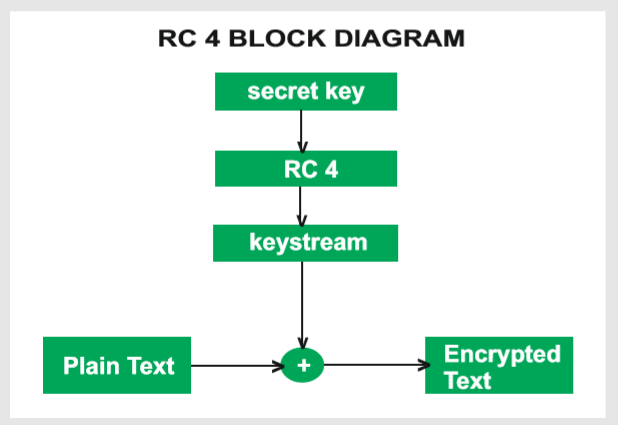Open in App
Not now

# What is RC4 Encryption?

• Difficulty Level : Hard
• Last Updated : 06 Dec, 2021

RC4 means Rivest Cipher 4 invented by Ron Rivest in 1987 for RSA Security. It is a Stream Ciphers. Stream Ciphers operate on a stream of data byte by byte. RC4 stream cipher is one of the most widely used stream ciphers because of its simplicity and speed of operation. It is a variable key-size stream cipher with byte-oriented operations. It uses either 64 bit or 128-bit key sizes. It is generally used in applications such as Secure Socket Layer (SSL), Transport Layer Security (TLS), and also used in IEEE 802.11 wireless LAN std.

### Why Encryption Is Important?

Unauthorized data access can be prevented by encryption. If we perform encryption then third parties can not have access to data which we share or receive. The encryption is done by using a secret key, or we can say that by using a public key and private key. Both sender and receiver are having their public key and private key through which encryption of plain text and decryption of ciphertext is performed.

### History of RC4 Encryption

RC4 was designed by Ron Rivest in 1987. He was working under RSA Security. Rivest Cipher 4 is an official name while it is also known as Ron’s Code. Initially, RC4 was trade secret but once it’s code spread in the public domain it was no more a trade secret. While Ron did not reveal the RC4 algorithm until 2014 when he described the history of RC4 in English Wikipedia.

### Applications of RC4

RC4 is used in various applications such as WEP from 1997 and WPA from 2003. We also find applications of RC4 in SSL from 1995 and it is a successor of TLS from 1999. RC4 is used in varied applications because of its simplicity, speed, and simplified implementation in both software and hardware.

### Types of RC4

There are various types of RC4 such as Spritz, RC4A, VMPC, and RC4A.

1. SPRITZ: Spritz can be used to build a cryptographic hash function, a deterministic random bit generator (DRBG), n an encryption algorithm that supports authenticated encryption with associated data (AEAD).
2. RC4A: Souraduyti Paul and Bart Preneel have proposed an RC4 variant, which they call RC4A, which is stronger than RC4.
3. VMPC: VMPC is another variant of RC4 which stands for Variably Modified Permutation Composition.
4. RC4A+: RC4A+ is a modified version of RC4 with a more complex three-phase key schedule which takes about three times as long as RC4 and a more complex output function which performs four additional lookups in the S array for each byte output, taking approximately 1.7 times as long as basic RC4.

### Algorithm

The algorithm operates on a user-selected variable-length key(K) of 1 to 256 bytes (8 to 2048 bits), typically between 5 and 16 bytes. To generate a 256-byte state vector S, the master key is used.
The first step is the array initialization. It is a character array of size 256 i.e. S. After that, for every element of the array, we initialize S[i] to i.

```Code for array initialization:
Char S;
int i;
for(i=0;i<256;i++)
S[i] = i
The array will look like -
S[] = {0, 1, 2, 3, ------, 254, 255}```

After this, we will run the KSA algorithm-

KSA is going to use the secret key to scramble this array. KSA is a simple loop, in which we are having two variable i and j. We are using these variables to rearrange the array. Rearranging the array is done by using a secret key.

```Code for KSA (Key Scheduling Algorithm ) :
int i, j=0;
for(i=0;i<256;i++)
{
j=( j + S[i] + T[i]) mod 256;
Swap(S[i], S[j]);
}```

KSA has been scrambled, S array is used to generate the PRGA(Pseudo Random Generation Algorithm). This is the actual Keystream.

```Code for PRGA ( Pseudo Random Generation Algorithm ):
i=j=0;
while(true)
{
i = ( i + 1 ) mod 256;
j = ( j + S[i] ) mod 256;
Swap( S[i], S[j] );
t = ( S[i] + S[j] ) mod 256 ;
k = S[t];
}```

This is the next step of scrambling.

### RC4 Block Diagram### Working of RC4

#### Encryption Procedure

1. The user inputs a plain text file and a secret key.
2. The encryption engine then generates the keystream by using KSA and PRGA Algorithm.
3. This keystream is now XOR with the plain text, this XORing is done byte by byte to produce the encrypted text.
4. The encrypted text is then sent to the intended receiver, the intended receiver will then decrypted the text and after decryption, the receiver will get the original plain text.

#### Decryption Procedure

Decryption is achieved by doing the same byte-wise X-OR operation on the Ciphertext.

Example: Let A be the plain text and B be the keystream (A xor B) xor B = A

1. RC4 stream ciphers are simple to use.
2. The speed of operation in RC4 is fast as compared to other ciphers.
3. RC4 stream ciphers are strong in coding and easy to implement.
4. RC4 stream ciphers do not require more memory.
5. RC4 stream ciphers are implemented on large streams of data.